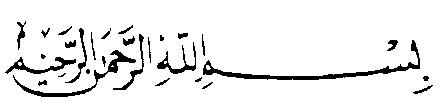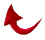View Original Document

Page - 1  -  2  -  3  -  4MUSLIM PERSPECTIVE

Bulletin of the UNITED ISLAMIC NATION

Published Monthly by Masjid Tucson

NOVEMBER 1988                                                         RABI ll 1409

MIRACLE OF QURAN EXPLODES

SOON OVERWHELMS THE WORLD

In the September issue of M.P., a great miracle was published. I reported the physical fact that the numbers mentioned in the Quran are 1, 2, 3, 4, 5, 6, 7, 8, 9, 10, 11, 12, 19, 20, 30, 40, 50, 60 ,70, 80, 99, 100, 200, 300, 1000, 2000, 3000, 5000, 50000, and 100000. The total of these numbers is 162146, or 19x8534. Anyone can easily verify, witness, and appreciate this miracle:

A deviation by 1 destroys the miracle.

In the October issue, I published the great discovery of Brother Abdullah Arik of Masjid Tucson. These new awesome facts authenticate every verse in the Quran. Here is how you can witness it:

(1) Sura 1 consists of 7 verses, add the verse numbers 1 + 2 + 3 + 4 + 5 + 6 + 7, and you get 28.

(2) Add the number of verses in the sura, 7, and you get 35.

(3) Write down the value for Sura 1 as 35.

(4) Do the same for Sura 2---it contains 286 verses. You add 1+2+3+ ... +286, and you get 41041.

(5) Add the number of verses in Sura 2 and you get (286+41041) 41327.

(6) Go on doing the same thing for every sura. The grand total is 339644, or 19x17876.

ANOTHER MIRACLE:

(7) Do the same calculations as described in # (1) above, but add the number of the sura. The value for Sura 1, therefore, becomes 36.

1. Repeat the same operation for all suras, you will find the grand total 346199, or 19x18221.

ANOTHER TRULY AWESOME FACT:

If you do these calculations [steps (7) and (8)] for the 29 initialed suras only [suras 2,3, 7, 10-15, 19, 20, 26-32, 36, 38, 40-46, 50,& 68], the grand total also is a multiple of 19, 190133, or 19x10007.

When you do the same calculations for the 85 un-initialed suras, the total comes to 19x8214, or 156066.

(9) Take the 29 initialed suras. Add the number of each sura to the number of initials in it. For example, Sura 2 is initialed with 3 initials, A.L.M., so you add 2+3=5.

(10) Add the number of verse(s) where the initials occur. The Quranic initials occur in Verse 1 in all initialed suras except one--Sura 42 has two sets of initials in 2 verses. The A.L.M. of Sura 2 occur in one verse, so you add 1 to the 5 and you get the final value 6 for Sura 2.

(11) Do this with every initialed sura. You guessed it: the grand total for all initialed suras is 931, or 19x49.

This phenomenal intricacy confirms that the initials of Sura 42 occur in 2 verses, and that Sura 68, contains 2 initials (NuN). So you think this is miraculous enough! Of course it is. But here is another truly astounding miracle. If you do the same calculations as in (9) thru (11), but multiply the sura number by the number of initials, instead of adding, the total is 2052, 19x108. ALLAHU AKBAR [Illustrations on Page 2]

HOT OFF THE COMPUTER

New Fantastic Discoveries

While writing Abdullah Arik's outstanding discoveries which are shown on Pages 1 and 2, Mr. Arik walked into my office with the following new findings.

These facts cover the 29 initialed suras:

(1) The first initialed sura is Sura 2: Add the sura number to the number of verses in this sura (2+286=288).

(2) Add the number of verses where the initials are found, 1, and you get 289.

(3) Add the geometrical values of the initials A.L.M. (1 + 30 + 40); you get 289+71=360.

(4) Repeat these same easy calculations for all 29 initialed suras

(5) The grand total is 7030, or 19x370.

ARE YOU READY FOR A SHOCK?

(6) Carry Out the calculations described above, but instead of adding the sura number plus the number of verses (2+286), multiply 2x286.

(7) Repeat same for all initialed suras.

(8) The grand total is 63536, or 19 x 3344, or 19 x 19x176. See illustrations below:

 Sura No. No. of Verses Initialed Verses Gematrical Value of the Initials Total Cumulative TOTAL 2 + 286 + 1 + 1+30+40 = 360 360 3 + 200 + 1 + 1+30+40 = 275 635 7 + 206 + 1 + 1+30+40+90 = 375 1010 - - - --- - - - 50 + 45 + 1 + 100 = 196 6809 68 + 52 + 1 + 50+50 = 221 7030=19x370

Note the multiplication signs in this table:

 Sura No. No. of Verses Initialed Verses Geometrical Value of the Initials Total Cumulative TOTAL 2 x 286 + 1 + 1+30+40 = 644 644 3 X 200 + 1 + 1+30+40 = 672 1316 7 X 206 + 1 + 1+30+40+90 = 1604 2920 50 x 45 + 1 + 100 = 2351 59899 68 x 52 + 1 + 50+50 = 3637 63536=19x19x176

Another Great Discovery:

If you multiply the geometrical value of the Quran's initials by the number initials, and add the number of initialed verses, the grand total is 10792, or 19x568.

 Sura No. Geometrical Value of its Initials No. of Initials Initialed Verses Total Cumulative Total 2 71 x 3 + 1 = 214 214 3 71 x 3 + 1 = 214 428 7 161 x 4 + 1 = 645 1973 - - - - - - 68 100 x 2 + 1 = 201 10792=19x568

ALL PARAMETERS OF THE INITIALED SURAS CONFIRMED

 Sura No. No. of Verses Initialed No. of Verses Geometrical Initials Value Grand Total Total 2 + 286 + 1 + 3 + 71 = 363 363 3 + 200 + 1 + 3 + 71 = 278 641 - 68 + 52 + 1 + 2 + 100 = 223 7109 2 x 286 x 1 x 3 x 71 = 121836 121836 3 x 200 x 1 x 3 x 71 = 127800 249636 - 68 x 52 x 1 x 2 x 100 = 707200 21455481

7109 + 21455481 = 21462590 = 19x1129610

MIRACLE OF THE QURAN EXPLODES

This table does not add the sura number:

 Sura No. Number of Verses Sum of Verse Number’s Total Cumulative Total 1 7 28 35 35 2 286 41041 41327 41362 9 127 8128 8255 131361 114 6 21 27 339644=19x17876

This table adds the sura number:

 Sura No. Number of Verses Sum of Verse Number’s Total Cumulative Total 1 7 28 36 36 2 286 41041 41329 41365 9 127 8128 8264 131406 114 6 21 141 346199=19x18221

This table covers the 29 initialed suras only:

 2 286 41041 41329 41329 3 200 20100 20303 61632 7 206 21321 21534 83166 50 45 1035 1130 188635 68 52 1378 1498 190133=19x10007

This table covers the 85 un-initialed suras:

 1 7 28 36 36 4 176 15576 15756 15792 9 127 8128 8264 48240 114 6 21 141 156066=19x8214

The following tables deal with the 29 initialed suras:

 Sura No. Number of Initials Initialed Verses Total Cumulative Total 2 + 3 1 6 6 3 + 3 1 7 13 50 + 1 1 52 860 68 + 2 1 71 931=19x49 2 x 3 1 7 7 3 x 3 1 10 17 50 x 1 1 51 1915 68 x 2 1 137 2052=19x108

ONE FINAL NAIL IN THE COFFIN OF

THE FALSE INJECTIONS 9:128-129

Take all the verses from the missing Bismillah (9:1) to the extra Bismillah (27:29):

(1) Add the sura number plus the number of verses in each sura.

(2) Of course, you use only 127 verses of sura 9, excluding the false injections 128 & 129, and you use only 29 verses from Sura 27 (since the Bismillah is found in Verse 30).

(3) Add the "Sum of Verse Numbers" (1 + 2+3+... + n, where n is the number of verses in the sura).

(4) You will find that the grand total is 119966, or 19x6314.

 Sura No. No. of Verses Sum of Verse Number’s Total Cumulative Total 9 + 127 + 8128 = 8264 8264 10 + 109 + 5995 = 6114 14378 26 + 227 + 25878 = 26131 119475 27 + 29 + 435 = 491 119966 (19x6314) Note: 9+10+11+12+13+14+15+16+17+18+19+20+21+22+23+24+25+26+27=342 (19x18)

None attains this except those who are extremely fortunate [41:35]

LETTERS FROM BELIEVERS ALL OVER THE WORLD

I am happily astonished at the number of true and courageous Muslims around the world who take the time to write down their support for God's messenger, and their commitment to worshiping Allah ALONE, and upholding His word, the Quran ALONE. Here is just one example from Pakistan:

Dear respected Rashad: Al hamdo Liliah! He has heard my prayers. Today I received and read the M.P.’s of July & Sept 88, and decided I too must write, as I had from long ago wanted to.

In the summer of 1971,I was discussing with a cousin the plight of the Muslims, at being un-Islamic and of different sects---l preferred to be just a Muslim--and said, 'If Allah Almighty would send a messenger (and why not now when we most need one) to unite & right these wrong-doer Muslims and other believers among 'Ahle Kitab. After I had said it I wondered if this could happen in my lifetime, and if I would have the courage to stand up and accept him. Yes Allah be praised, He has in my lifetime, blessed me to be one to witness and accept you and your teachings of the Quran in its pure and clear sense.Return to Table of Contents